# Projectile Motion Calculator

Created by Bogna Szyk and Hanna Pamuła, PhD
Reviewed by Steven Wooding and Jack Bowater
Last updated: Dec 22, 2022

Our projectile motion calculator is a tool that helps you analyze parabolic projectile motion. It can find the time of flight, but also the components of velocity, the range of the projectile, and the maximum height of flight. Continue reading if you want to understand what projectile motion is, get familiar with the projectile motion definition, and determine the abovementioned values using the projectile motion equations.

Prefer watching over reading? Learn all you need in 90 seconds with this video we made for you:

## What is projectile motion? Projectile motion definition

Imagine an archer sending an arrow in the air. It starts moving up and forward, at some inclination to the ground. The further it flies, the slower its ascent is – and finally, it starts descending, moving now downwards and forwards and finally hitting the ground again. If you could trace its path, it would be a curve called a trajectory in the shape of a parabola. Any object moving in such a way is in projectile motion. By the way, we have the arrow speed calculator that analyzes the motion of arrows - give it a try!

Only one force acts on a projectile – the gravity force. Air resistance is always omitted. If you drew a free body diagram of such an object, you would only have to draw one downward vector and denote it “gravity”. If there were any other forces acting on the body, then – by projectile motion definition – it wouldn't be a projectile.

## Projectile motion analysis

Projectile motion is pretty logical. Let's assume you know the initial velocity of the object $V$, the angle of launch $\alpha$, and the initial height $h$. Our projectile motion calculator follows these steps to find all remaining parameters:

1. Calculate the components of velocity.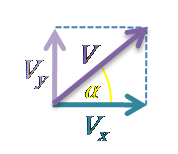• The horizontal velocity component $V_\mathrm x$ is equal to $V \cos\alpha$.
• The vertical velocity component $V_\mathrm y$ is equal to $V \sin\alpha$.
• Three vectors - $V$, $V_\mathrm x$ and $V_\mathrm y$ - form a right triangle.

If the vertical velocity component is equal to 0, then it's the case of horizontal projectile motion. If, additionally, α = 90°, then it's the case of free fall. We tackled both problems in the horizontal projectile motion calculator and free fall calculator, respectively.

1. Write down the equations of motion.

Distance

• Horizontal distance traveled can be expressed as $x = V_\mathrm x t$ where $t$ is the time.
• Vertical distance from the ground is described by the formula $y = h + V_\mathrm y t - g t^2 / 2$, where $g$ is the gravity acceleration.

Velocity

• Horizontal velocity is equal to $V_\mathrm x$.
• Vertical velocity can be expressed as $V_\mathrm y - g t$.

Acceleration

• Horizontal acceleration is equal to 0.
• Vertical acceleration is equal to $-g$ (because only gravity acts on the projectile).
1. Calculate the time of flight.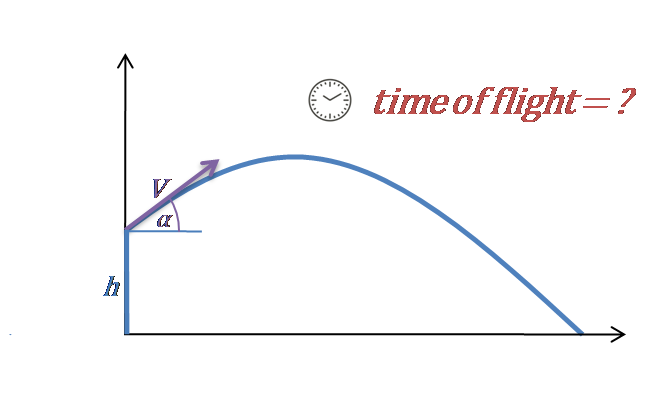• Flight ends when the projectile hits the ground. We can say that it happens when the vertical distance from the ground is equal to 0. In the case where the initial height is 0, the formula can be written as: $V_\mathrm y t - g t^2 / 2 = 0$. Then, from that equation, we find that the time of flight is
$\quad t = 2 \frac{V_\mathrm y}{g} = 2 \frac{V}{g} \sin\alpha.$
• However, if we're throwing the object from some elevation, then the formula is not so nicely reduced as before, and we obtain a quadratic equation to solve: $h + V_\mathrm y t - g t^2 / 2 = 0$. After solving this equation, we get:
$t \! = \! \frac{V \sin\alpha \! + \! \sqrt{ \! (V \sin\alpha)^2 \! + \! 2 g h}}{g}$
1. Calculate the range of the projectile.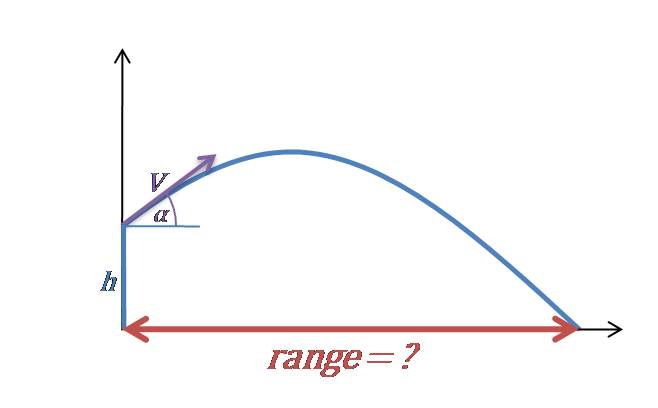• The range of the projectile is the total horizontal distance traveled during the flight time. Again, if we're launching the object from the ground (initial height = 0), then we can write the formula as $R = V_\mathrm x t = V_\mathrm x \times 2 \times V_\mathrm y / g$. It may be also transformed into the form: $R = V^2 \sin(2\alpha) / g$

• Things are getting more complicated for initial elevation differing from 0. Then, we need to substitute the long formula from the previous step as $t$:

$R = V_\mathrm x t = \\[0.8em] V \! \cos \alpha \frac{V \! \sin\alpha \! + \! \sqrt{ \! (V \! \sin\alpha)^2 \! + \! 2 g h}}{g}$
1. Calculate the maximum height.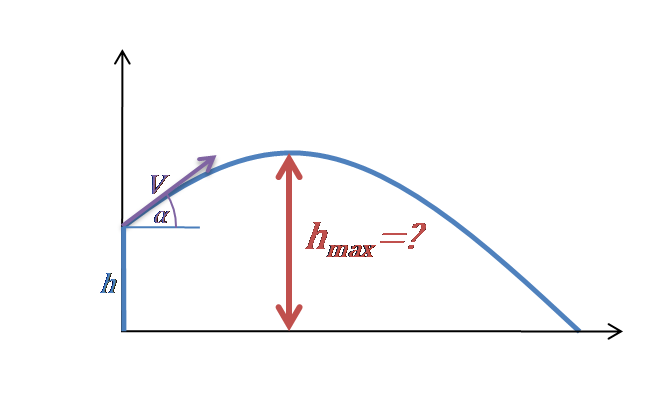• When the projectile reaches the maximum height, it stops moving up and starts falling. It means that its vertical velocity component changes from positive to negative – in other words, it is equal to 0 for a brief moment at time $t(V_\mathrm y=0)$.
• If $V_\mathrm y - g t(V_\mathrm y=0) = 0$, then we can reformulate this equation to $t(V_\mathrm y=0) = V_\mathrm y / g$.
• Now, we simply find the vertical distance from the ground at that time:
$\begin{split} h_\mathrm{max} = &V_\mathrm y t( V_\mathrm y \! = \! 0) \! - \! \frac{g t^2 (V_\mathrm y \! = \! 0)}{2} = \\[0.8em] &\frac{V^2_\mathrm y}{2 g} = \frac{V^2 \sin ^2 \alpha}{2 g} \end{split}$
• Fortunately in the case of launching a projectile from some initial height $h$, we need to simply add that value into the final formula:
$h_\mathrm{max} = h + \frac{V^2 \sin^2 \alpha}{2 g}$

## Projectile motion equations

Uff, that was a lot of calculations! Let's sum that up to form the most essential projectile motion equations:

1. Launching the object from the ground (initial height h = 0)
• Horizontal velocity component: $V_\mathrm x = V \cos \alpha$
• Vertical velocity component: $V_\mathrm y = V \sin \alpha$
• Time of flight: $t = 2 V_\mathrm y / g$
• Range of the projectile: $R = 2 V_\mathrm x V_\mathrm y / g$
• Maximum height: $h_\mathrm{max} = V^2_\mathrm y / (2 g)$
1. Launching the object from some elevation (initial height h > 0)
• Horizontal velocity component: $V_\mathrm x = V \cos \alpha$
• Vertical velocity component: $V_\mathrm y = V \sin \alpha$
• Time of flight: $t = \left[V_\mathrm y + \sqrt{V^2_\mathrm y + 2 g h}\right] / g$
• Range of the projectile: $R = V_\mathrm x \left[V_\mathrm y + \sqrt{V^2_\mathrm y + 2 g h}\right] / g$
• Maximum height: $h_\mathrm{max} = h + V^2_ \mathrm y / (2 g)$

Using our projectile motion calculator will surely save you a lot of time. It can also work 'in reverse'. For example, enter the time of flight, distance, and initial height, and watch it do all calculations for you!

Be sure also to check the parabola calculator to learn more about such a curve from a mathematical point of view.

## FAQ

### Does projectile motion have to travel horizontally?

No, projectile motion and its equations cover all objects in motion where the only force acting on them is gravity. This includes objects that are thrown straight up, those thrown horizontally, those that have a horizontal and vertical component, and those that are simply dropped.

### What is an example of projectile motion?

Objects with projectile motion include: keys being thrown, a 300 kg projectile being thrown 90 m by a trebuchet, a football being kicked so that it no longer touches the ground, a diver jumping from a diving board, an artillery shell the moment it leaves the barrel, and a car trying to jump a bridge.

### How can a projectile fall around the Earth?

There is only one force acting on a projectile - gravity. This means that an object will eventually fall to Earth. But what about if the object is moving so fast horizontally that, by the time it reaches the ground, the ground is no longer there? This is the principle that governs satellites.

### How do I find acceleration in projectile motion?

There is only one force acting on an object in projectile motion - gravity. This means that any change in vertical speed is due to gravitational acceleration, which is 9.81 m/s2 (32.2 ft/s2) on Earth. In the horizontal direction, there is no change in speed, as air resistance is assumed to be negligible, so acceleration is 0.

### What factors affect the motion of a projectile launched horizontally?

Initial velocity, the initial height the projectile is being launched from, and gravity will all affect a projectile launched horizontally. Air resistance will also have an effect in real life, but for most theoretical calculations it is negligible and is therefore ignored. If the projectile has wings, this will also impact its motion, as it will glide.

### What exactly is a projectile?

A projectile is an object that is in motion, in the air and has no force acting upon it other than the acceleration due to gravity (this means that it cannot be self-propelled). You can probably think of many examples: a thrown ball or a stone thrown from a trebuchet. Even the Moon is a projectile, with respect to the Earth!

### What are the characteristics of projectile motion?

The properties of projectile motion are that the object’s horizontal velocity does not change, that it’s vertical velocity constantly changes due to gravity, that the shape of its trajectory will be a parabola, and that the object is not affected by air resistance.

### Who first accurately described projectile motion and when?

Galileo was the first person to describe projectile motion accurately, by breaking down motion into a horizontal and vertical component, and realizing that the plot of any object's motion would always be a parabola. He described it in his book, On Motion, published around the 1590s.

### Why does a projectile follow a curved path?

An object follows a parabola because of how its two components of motion - the horizontal and vertical - are affected by gravity. The horizontal component is not affected by gravity at all, and so changes in a constant, linear fashion. The vertical part, however, is constantly affected by gravity, and so it will increase in height, and then decrease, accelerating due to gravity.

### Why is 45 degrees the optimal angle for projectiles?

The equation for the distance traveled by a projectile being affected by gravity is sin(2θ)v2/g, where θ is the angle, v is the initial velocity and g is acceleration due to gravity. Assuming that v2/g is constant, the greatest distance will be when sin(2θ) is at its maximum, which is when 2θ = 90 degrees. This means θ = 45 degrees.

Bogna Szyk and Hanna Pamuła, PhD
Initial parameters
Initial velocity (V)
ft/s
Angle of launch (α)
deg
Initial height (h)
ft
Time of flight (t)
sec
Distance (d)
ft
Maximum height (hmax)
ft
Flight parameters at given time
Time
sec
Velocity
ft/s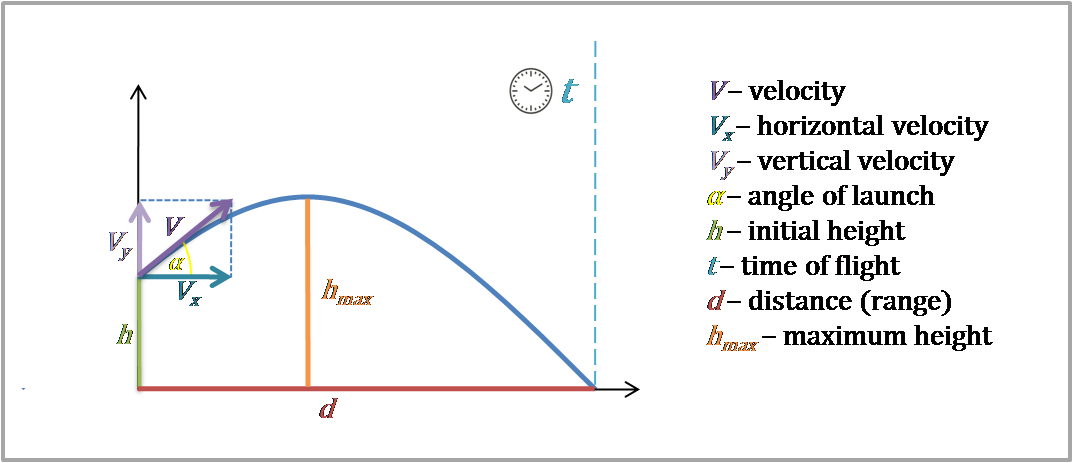People also viewed…

### Black hole collision

The Black Hole Collision Calculator lets you see the effects of a black hole collision, as well as revealing some of the mysteries of black holes, come on in and enjoy!

### Photon detection efficiency (SiPM)

Photon detection efficiency calculator is a handy tool that allows for a quick conversion between responsivity and Photon Detection Efficiency (PDE) in Silicon Photomultipliers (SiPMs).

### Resultant force

Use the net force calculator to find the resultant force on a body.

### Secretary problem (Valentine's day)

Use the dating theory calculator to enhance your chances of picking the best lifetime partner.